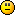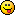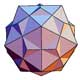# Math Is Fun Forum

Discussion about math, puzzles, games and fun.   Useful symbols: ÷ × ½ √ ∞ ≠ ≤ ≥ ≈ ⇒ ± ∈ Δ θ ∴ ∑ ∫  π  -¹ ² ³ °

You are not logged in.

## #1 2009-04-12 09:19:13

JaneFairfax
MemberRegistered: 2007-02-23
Posts: 6,868

### Permutations

A permutation is a bijection from the set
to itself. The set of all permutations forms a group of order
under composition of mappings, called the symmetric group of degree
and denoted
.

Permutations may be odd or even. Here is the most intuitive definition I can come up with for odd and even permutations. It is not the definition given in John F. Humphreyss book A Course in Group Theory, but it will do.

Given

, let

Then we say that

is even if the number of elements in
is even, and odd if
is odd.Last edited by JaneFairfax (2009-04-14 05:13:43)

Offline

## #2 2009-04-12 09:42:27

JaneFairfax
MemberRegistered: 2007-02-23
Posts: 6,868

### Re: Permutations

Note that

Hence the identity is always an even permutation.

Also

A transposition

(where
) is a permutation which maps
to
and vice versa, leaving all other numbers fixed. Consider the ordered pairs

Under the transposition

, the image of the first component of each ordered pair is
, which is greater than the second component of each ordered pair (which is fixed in the transposition)

Hence

.

Similarly, the ordered pairs

are in

, since the transposition maps the second component to
while fixing the first component.

The only other ordered pair that can belong to

is
.

, which is odd.Offline

## #3 2009-04-12 09:58:59

JaneFairfax
MemberRegistered: 2007-02-23
Posts: 6,868

### Re: Permutations

This definition of odd/even permutations is at least intuitive. Unfortunately it is also mathematically unwieldy. Try proving, using this definition, that the composition of two even permutations is an even permutation, for instance.Humphreyss definition, in terms of properties of an n-variable polynomial (which I incidentally recognize as the determinant of the Vandermonde matrix), while not so intuitive, is more amenable to mathematical manipulation. Under this more flexible definition, the set of even permutations of S[sub]n[/sub] arises naturally as the kernel of a homomorphism from S[sub]n[/sub] to a 2-element group. This is thus a subgroup of index 2, called the alternating group A[sub]n[/sub]  and it follows without more ado that the product of two even permutations is even.Offline

## #4 2009-04-12 10:20:36

JaneFairfax
MemberRegistered: 2007-02-23
Posts: 6,868

Offline

## #5 2010-11-29 02:13:44

JaneFairfax
MemberRegistered: 2007-02-23
Posts: 6,868

### Re: Permutations

Okay, here is another definition of even and odd permutations.

[align=center]

[/align]

Lets look at the expression on the right-hand side. The denominator is a product of

factors, and so is the numerator. Let
be a factor in the denominator. Either
or
. In the former case
is a factor in the numberator, while in the latter case
is a factor in the numerator. Conversely, if
is a factor in the numerator, then (as
) either
is a factor in the denominator (if
) or
is a factor in the denominator (if
).

So we see that if

is a factor in the denominator then either
or
is a factor in the numerator and conversely if
is a factor in the numerator then either
or
is a factor in the denominator. It follows that
is always either +1 or −1.
We define
to be even if
is and odd if
.

Last edited by JaneFairfax (2010-11-30 05:50:12)

Offline

## #6 2010-11-30 05:25:23

JaneFairfax
MemberRegistered: 2007-02-23
Posts: 6,868

### Re: Permutations

[align=center]

[/align]

so we need to show that

.

We first rewrite some of the factors in this product:

Note that this is equivalent to multiplying by

which means that it leaves the value of the product unchanged. Doing this for all i, j, we can ensure that all the factors in the denominator are of the form
for
, with the corresponding factors in the numerator the same way round. Hence

as required.

Last edited by JaneFairfax (2010-11-30 05:26:35)

Offline

## #7 2010-12-06 07:55:05

JaneFairfax
MemberRegistered: 2007-02-23
Posts: 6,868

### Re: Permutations

JaneFairfax wrote:

[align=center]

[/align]

so we need to show that

.

We first rewrite some of the factors in this product:

Note that this is equivalent to multiplying by

which means that it leaves the value of the product unchanged. Doing this for all i, j, we can ensure that all the factors in the denominator are of the form
for
, with the corresponding factors in the numerator the same way round. Hence

as required.

Offline

## #8 2011-07-15 20:45:34

Chalisque
MemberRegistered: 2011-07-15
Posts: 6

### Re: Permutations

The feeling of stability that you get with a tripod is present with an even permutation but not with an odd one (and this does not depend upon any significant subtleties of your arithmetic or set-theoretic foundations) so the trick to getting a student to intuitively understand the difference between odd and evenness is to lead them somehow to this feeling, and the path is potentially different for every student since no two people start from the same point of view.

chalisque.com/dr

Offline